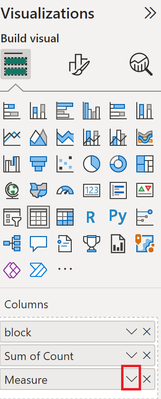cancel
Showing results for
Did you mean:Helper III

## If result return "Not Started", then text is red color

Hi ...

Below is my measure :

Weighted Achievement (%) =

VAR Score = SUM('kpidb kpi_submissions'[score])
VAR Weightage = SUM('kpidb kpis'[weightage])
VAR Stage = 3
VAR Result = Score / Stage * Weightage
RETURN
IF(ISBLANK(Result), "Not Started", Result)

How can we return if the result equal to "Not Started" then the text should also in red color ....

Thank you

Regards,
NickzNickz

1 ACCEPTED SOLUTIONSuper User

Hi @NickzNickz ,

You could create an additional measure which looks like this:

`ColourCodeMeasure = If ( [Measure] = "hello", "red", BLANK() )`

Here the result for my mockup data:Your measure would be something like this. Note you could even use HEX number instead of "red":

`ColourCodeMeasure = If ( [Weighted Achievement (%)] = "Not Started", "red", BLANK() )`

Then click on the arrow facing down in the visualization pane > Conditional Formatting > Font ColorChoose the following settings:Let me know if this helps 🙂

Proud to be a Super User!

2 REPLIES 2Super User

Hi @NickzNickz ,

You could create an additional measure which looks like this:

`ColourCodeMeasure = If ( [Measure] = "hello", "red", BLANK() )`

Here the result for my mockup data:Your measure would be something like this. Note you could even use HEX number instead of "red":

`ColourCodeMeasure = If ( [Weighted Achievement (%)] = "Not Started", "red", BLANK() )`

Then click on the arrow facing down in the visualization pane > Conditional Formatting > Font ColorChoose the following settings:Let me know if this helps 🙂

Proud to be a Super User!Helper III

Really appreciate it... The result is as expected.... Thanks a lot ...

Regards,

NickzNickz